# The Ever so Lovely Bézier Curve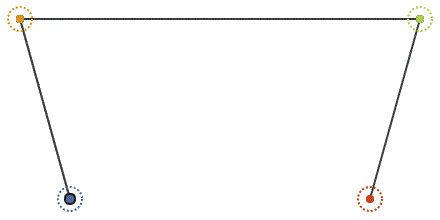Cubic Bézier with intermediate points & lines

Bézier curves are, to me, one of the best examples of mathematical beauty. It’s fascinating what such a simple function on some points can achieve. Below is a step-by-step visualization of how a cubic Bézier curve can be constructed using simple rules on points and lines. Note each point’s position along its own line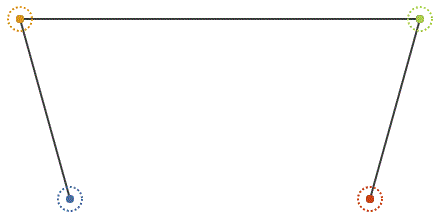Step-by-step construction of a cubic Bézier

If you look closely, you may see we’re using Linear interpolation, also known as lerp! Lerping between each set of original points, and then between the resulting points, gives rise to curves. Looking at the second set of points — we can see that even they themselves form two quadratic Bézier curves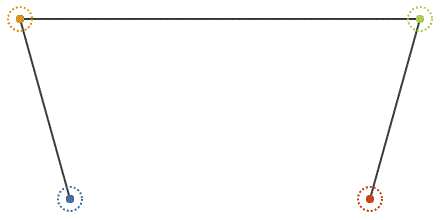Quadratic Bézier curves appear halfway in constructing a cubic Bézier

How does it work in code?
To get a point in the curve, you simply provide a value between 0 and 1, known as the t-value, to this set of lerps:

`a = lerp( p0, p1, t );b = lerp( p1, p2, t ); c = lerp( p2, p3, t ); d = lerp( a, b, t );e = lerp( b, c, t ); point = lerp( d, e, t );`

While the code above is easy to understand, there are computationally more efficient, though less numerically stable, ways of evaluating this. If you work out the math behind all lerps, and simplify, you end up with four equations that act as weights on each point.

Now let’s look at our Bézier evaluation again, this time with lerps expressed as math:

`a = (1-t)p0 + tp1b = (1-t)p1 + tp2c = (1-t)p2 + tp3d = (1-t)a + tbe = (1-t)b + tcpoint = (1-t)d + te`

Let’s collapse it all into a single equation, creating this monster:

`point = (1-t)((1-t)((1-t)p0+tp1)+t((1-t)p1+tp2)) + t((1-t)((1-t)p1+tp2)+t((1-t)p2+tp3))`

Then expand to untangle all points and t values:

`point =-p0t³ + 3p0t² — 3p0t + p0 +3p1t³ — 6p1t² + 3p1t -3p2t³ + 3p2t² +p3t³`

If you look at each line here, you can see that each belong to a specific point. With that in mind, we can finally simplify it by separating out each point as a lone factor:

`point =p0(-t³+3t²-3t+1) +p1(3t³-6t²+3t) +p2(-3t³+3t²) +p3(t³)`

And thus, we now have what is called basis functions, which happen to be called Bernstein polynomials, for our cubic Bézier curve :)
We can visualize this version as 4 vectors being added together, with varying lengths depending on what the t-value evaluates to on each corresponding basis function: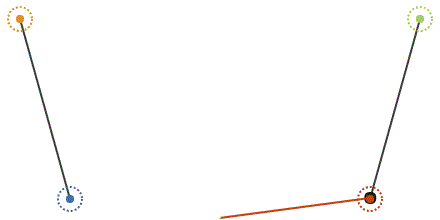Construction using the weighted sum of each point, using basis functions

A quirk when using Bézier curves is that the t-value is actually not the same thing as percentage of distance along the curve. The difference in t-value between each segment here is constant, yet the distance, is not. The animation is linearly animating t, yet the speed is not constant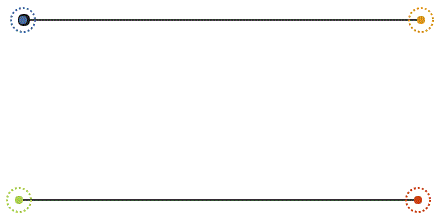Linearly animating t, showcasing the non-uniform spatial distance between t-values

This is often a problem for animation. One solution to this, is to build a lookup table that can help you convert percentage along the spline to t.

The basic idea is to construct a table containing the cumulative distance for each sampled point, where t is incremented uniformly (Slide 75). Then in order to convert from percentage to t, we first need the desired distance along the spline. We get this simply by multiplying the percentage by the total length of the curve, which is going to be the last entry of the length table.

We then iterate through the table, to find at what index we reach the desired distance. The distance is likely to land between two values in the length table, so we simply do an inverse lerp / remap, from where in the distance table it would be, converting it back to the t-values of each index, interpolating between them. How does it look when finished? Well, uniform, like this!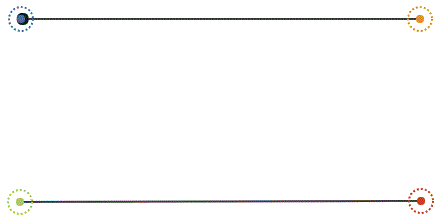Animating with uniform speed using a lookup table

Want to play with curves? Give it a try!
❱ acegikmo.com/bezier

Want to ask questions? Follow live development of a game using Bézier curves in its level editor? Feel free to follow me over on Twitch! I’m always open for questions of any kind, regularly talking about code, math and game dev!
twitch.tv/acegikmo

That’s all for now — thanks for reading ❤️